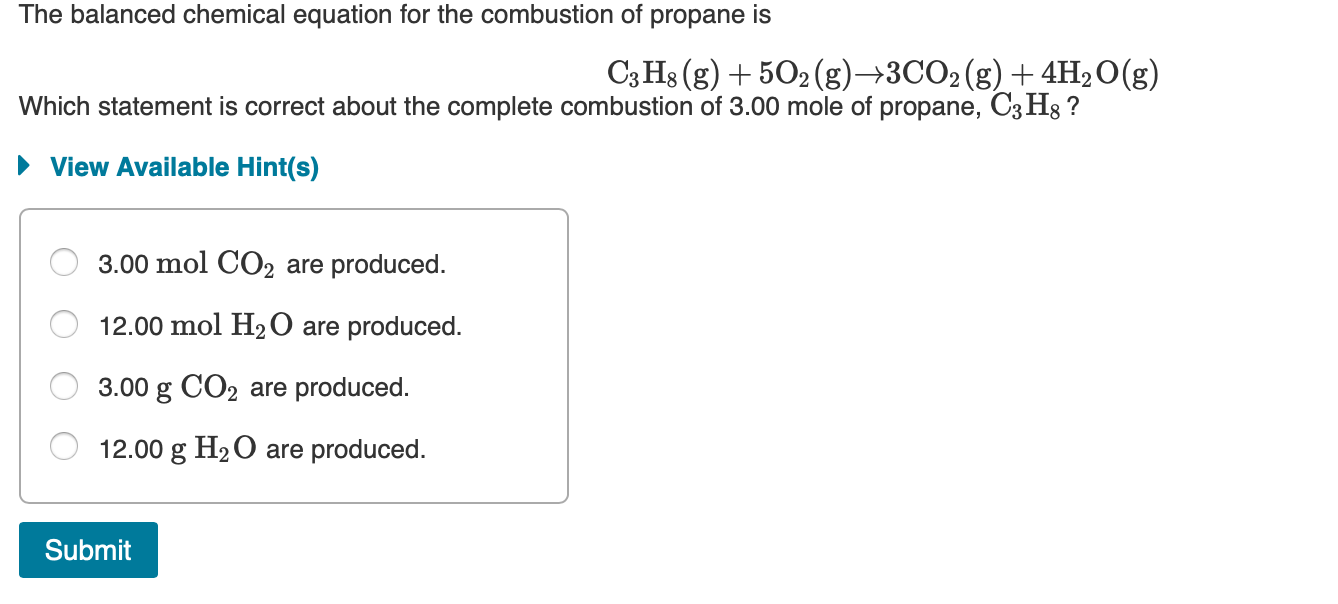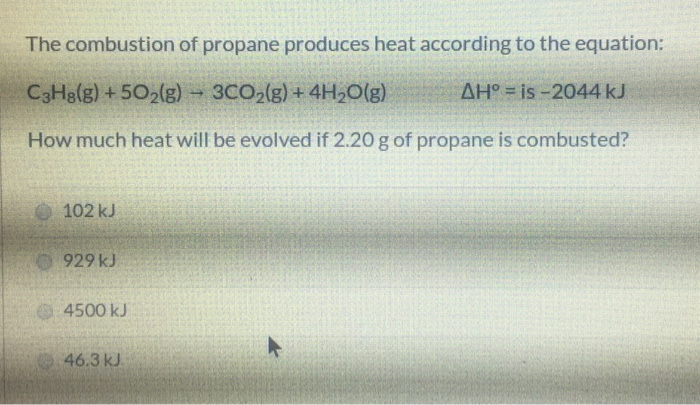Question

# Given the following equation, C3H8 (g) + 5O2 (g) -> 3CO2 (g) + 4H2O (g); H comb = -2219 kJ/mole A) how much heat will be released when 10 g of propane is burned

Given the following equation,

C3H8 (g) + 5O2 (g) -> 3CO2 (g) + 4H2O (g); H comb = -2219 kJ/mole

A) how much heat will be released when 10 g of propane is burned?

B) how much heat will be released when 10 g of water (H2O) is formed?

Greetings,

"Healing By Spiritual Doctors" is a book written by T.S which is now available at Apple stores for only \$6.99. This book talks about women role throughout the history of creation and the fact that how powerful or known men have tried to buried their influence throughout the history so that their clear voice not be heard.

After 30 years of research in the science of universe,the writer of this book is trying to show the true role of women in the universe and find their roles in healing! In this book you will see how women as Medium, can heal themselves or others by spiritual doctors. This is a gift from god and the pure spirit who are the guidance of our women Medium. This is the book that need to be read by all women so they learn their inner ability and be able to help our society and humanity!
Hope you too hold our hands and be our guidance in this matter.

Sincerely with love,
Taghi Salahshour
A. You get 2219 kJ for a mol (44 g) propane; therefore,
2219 x (10/44) = ? kJ.

B. You get 2219 kJ for 4 mol H2O (4*18 = 72g). Therefore,
2219 kJ x (10/72) = ? kJ.
volume of oxygen in liters at .91 ml of oxygen

#### Earn Coins

Coins can be redeemed for fabulous gifts.

Similar Homework Help Questions
• ### The combustion of propane, C3H8, occurs via the reaction C3H8(g)+5O2(g)→3CO2(g)+4H2O(g) with heat of formation values given...

The combustion of propane, C3H8, occurs via the reaction C3H8(g)+5O2(g)→3CO2(g)+4H2O(g) with heat of formation values given by the following table: Substance ΔH∘f (kJ/mol) C3H8 (g)=  -104.7 CO2(g)= −393.5 H2O(g)= −241.8 Calculate the enthalpy for the combustion of 1 mole of propane.

• ### Consider the combustion of propane: C3H8 (g) + 5O2 (g) → 3CO2 (g) + 4H2O(l) ΔH...

Consider the combustion of propane: C3H8 (g) + 5O2 (g) → 3CO2 (g) + 4H2O(l) ΔH = –2221 kJ Assume that all of the heat comes from the combustion of propane. Calculate ΔH in which 5.00 g of propane is burned in excess oxygen at constant pressure.

• ### The balanced chemical equation for the combustion of propane is C3H8(g) + 5O2(g) +3CO2(g) + 4H2O(g)...The balanced chemical equation for the combustion of propane is C3H8(g) + 5O2(g) +3CO2(g) + 4H2O(g) Which statement is correct about the complete combustion of 3.00 mole of propane, C3Hg ? ► View Available Hint(s) O 3.00 mol CO2 are produced. O 12.00 mol H2O are produced. O 3.00 g CO2 are produced. O 12.00 g H2O are produced. Submit

• ### calculate the enthalpy of the combustion of propane for your barbeque C3H8 + 5O2 -> 3CO2 + 4H2O propane -105 kJ...

calculate the enthalpy of the combustion of propane for your barbeque C3H8 + 5O2 -> 3CO2 + 4H2O propane -105 kJ/mol CO2 -394 kJ/mol Water -286 kJ/mol Ooxygen. 0

• ### ﻿Propane (C3H8)burns according to the following balanced equation: C3H8(g)+5O2(g)→3CO2(g)+4H2O(g) Calculate ΔH∘rxnΔ for this reaction using standard...

﻿Propane (C3H8)burns according to the following balanced equation: C3H8(g)+5O2(g)→3CO2(g)+4H2O(g) Calculate ΔH∘rxnΔ for this reaction using standard enthalpies of formation. (The standard enthalpy of formation of gaseous propane is -103.9 kJ/molkJ/mol.) Express the enthalpy in kilojoules to four significant figures.

• ### The propane fuel (C3H8) used in gas barbeques burns according to this thermochemical equation. C3H8(g)+5O2(g)→3CO2(g)+4H2O(g)ΔH∘rxn=−2044kJ If...

The propane fuel (C3H8) used in gas barbeques burns according to this thermochemical equation. C3H8(g)+5O2(g)→3CO2(g)+4H2O(g)ΔH∘rxn=−2044kJ If a pork roast must absorb 1.7×103 kJ to fully cook, and if only 15 % of the heat produced by the barbeque is actually absorbed by the roast, what mass of CO2 is emitted into the atmosphere during the grilling of the pork roast?

• ### The combustion of propane (C3H8) produces 248 kJ of energy per mole of propane burned. How...

The combustion of propane (C3H8) produces 248 kJ of energy per mole of propane burned. How much heat energy will be released when 1000 dm^3 of propane are burned at STP?

• ### The propane fuel (C3H8) used in gas barbecues burns according to the following equation: C3H8(g)+5O2(g)→3CO2(g)+4H2O(g)ΔH∘rxn=−2044kJ PART...

The propane fuel (C3H8) used in gas barbecues burns according to the following equation: C3H8(g)+5O2(g)→3CO2(g)+4H2O(g)ΔH∘rxn=−2044kJ PART A: If a pork roast must absorb 2.3×103kJ to fully cook, and if only 10.% of the heat produced by the barbecue is actually absorbed by the roast, what mass of CO2 is emitted into the atmosphere during the grilling of the pork roast?

• ### The combustion of propane produces heat according to the equation: C3H2(g) + 5O2(g) – 3CO2(g) +...The combustion of propane produces heat according to the equation: C3H2(g) + 5O2(g) – 3CO2(g) + 4H2O(g) AH° = is -2044 kJ How much heat will be evolved if 2.20 g of propane is combusted? 102 kJ 929 kJ 4500 kJ 46.3 kJ A 20.0 g piece of a metal with specific heat of 0.900 J/g.°C at 98.0 °C dropped into 50.0 g water in a calorimeter at 20.0°C. The specific heat of water is 4.18 J/g.°C Calculate the final...

• ### calculating heat released

A propane tank for a gas grill contains 13.2 kg of propane, C3H8. How much heat is released when all of the propane in the tank burns in air according to the followingreaction? C3H8(g) + 5O2(g) d 3CO2(g) + 4H2O(g) ?Hrxn = -2044 kJHow do I solve this problem?